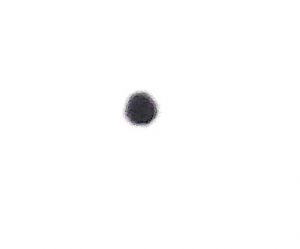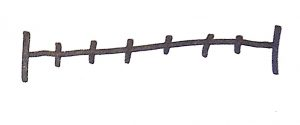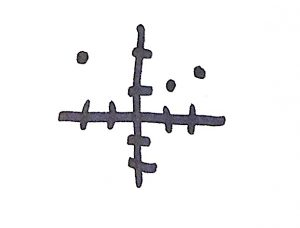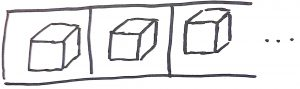# Problem Analysis: High Dimensional Spaces

April 27, 2017Previously we were discussing our how you probably want your valid input blob to be a metric space.  Let’s move on to another aspect that can make a problem difficult to work with.  This can apply to either the valid input blob (I) or the output blob (II).  We want our blobs to be low dimensional spaces.  The lower the better.  Let’s take a look at an example.

``public void Zero() { ... } public void One( int i ) { ... } public void Two( int x, int y ) { ... } public void Three( int x, int y, char c ) { ... }``

If you’re thinking about functions, then the dimensions may be how many parameters the function is taking.  However any given parameter can also be a multidimensional object, so you also have to consider what kind of parameters you are passing in.

``public void Function( int i, string s, string[] words ) { ... }``

The intuition has an easier time dealing with lower dimensional items.  This is mostly because there’s less information to have to keep track of.  Additionally, similar to metric spaces, low dimensional spaces are easier for the brain’s natural spatial processing to deal with because we train our whole lives to deal with a physical world that is mostly composed of only a few dimensions.Zero dimensional spaces are great.  There’s only one thing and that’s the end of the story.  Really the only question is whether or not it’s present.  In practice it’s pretty rare to actually bump into these things, but it’s a good anchor point.  This is about as simple as things can get.One dimensional spaces are also pretty easy to think about.  These are things like integers.Two and three dimensional spaces start to hit the limit of what is easy to mentally visualize.  We can still sort of graph what’s happening on paper, but it starts to become more of a chore.Four dimensional spaces is where we first start to really run into problems with our intuition.  You can sort of graph it as a three dimensional space with a time dimension.  This isn’t too bad if your space is modeling something like the physical world.  Unfortunately, for an arbitrary function it isn’t obvious which dimension should be the time dimension.  Also for exotic parameters jumping from one time slice to the next might cause radical changes to the three dimensional space component.  Instead of seeing a series of cubes, you might observe a cube, then a scatter plot, then a triangle, then a sphere, etc.  Instead of being able to quickly take a look at your space and understand its nature, you have to think about it for a while.  And then still miss an important detail.

However, the situation actually gets worse.  Consider the volume of an n-ball.  As the number of dimensions gets higher, the coefficient that you multiply the radius of the n-ball by gets smaller.  If you take a look at the generalized equation, you’ll see that the coefficient grows smaller much faster than the radius grows larger.  So, if you embed a high dimensional n-ball inside of a high dimensional n-cube such that the diameter of the n-ball is equal to the length of the n-cube, then the n-cube turns out to have a vastly larger volume than the n-ball.  There’s more volume in the corners of a high dimensional n-cube than there are in its center?  And this is just a simple space like a cube and a sphere … what implications does this have when you have five different arrays each filled with exotic data structures all being sent into the same function?

### High dimensional blobs make problems difficult because they baffle your intuition in sometimes very surprising ways.

Next time:  Topological holes.